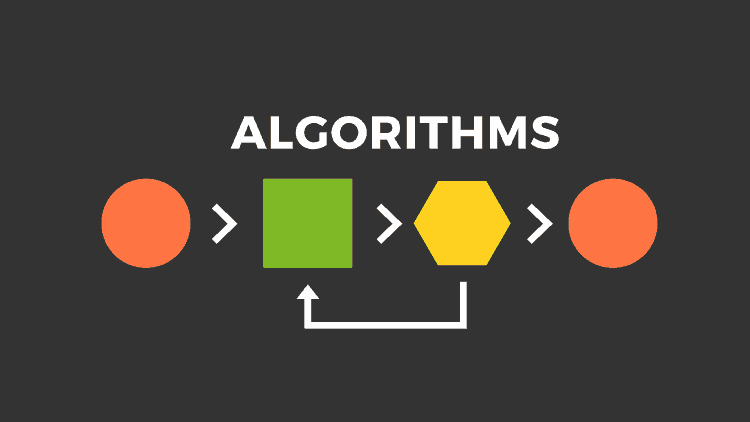# Learning Python with Advent of Code Walkthroughs

Dazbo's Advent of Code solutions, written in Python

# The Python Journey - Useful Algorithms## Overview

I’ve written a bunch of algorithms which I find useful and reusable…

## Merging Overlapping Intervals

``````def merge_intervals(intervals: list[List]) -> list[list]:
""" Takes intervals in the form [[a, b][c, d][d, e]...]
Intervals can overlap.  Compresses to minimum number of non-overlapping intervals. """
intervals.sort()
stack = []
stack.append(intervals)

for interval in intervals[1:]:
# Check for overlapping interval
if stack[-1] <= interval <= stack[-1][-1]:
stack[-1][-1] = max(stack[-1][-1], interval[-1])
else:
stack.append(interval)

return stack

intervals = [[6, 8], [1, 9], [2, 4], [4, 7]]
merged = merge_intervals(intervals)
print(merged)
``````

Output:

``````[[1, 9]]
``````

Here I’ve created a generic binary search algorithm that can be used to pass a candidate number to an arbitrary function, and to finish when the function returns our goal.

``````def binary_search(target, low:int, high:int, func, *func_args, reverse_search=False) -> int:
""" Generic binary search function that takes a target to find,
low and high values to start with, and a function to run, plus its args.
Implicitly returns None if the search is exceeded. """

res = None  # just set it to something that isn't the target
candidate = 0  # initialise; we'll set it to the mid point in a second

while low < high:  # search exceeded
candidate = int((low+high) // 2)  # pick mid-point of our low and high
# print(f"{candidate}->{res}")
res = func(candidate, *func_args) # run our function, whatever it is
if res == target:
return candidate  # solution found

comp = operator.gt if not reverse_search else operator.lt
if comp(res, target):
low = candidate
else:
high = candidate
``````

## Get Factors

Here is a function that returns all the factors for a given integer. Note how I’m making use of the `cache` decorator, in order to cache the factors of any integer that has been seen before.

``````@cache
def get_factors(num: int) -> set[int]:
""" Gets the factors for a given number. Returns a set[int] of factors.
# E.g. when num=8, factors will be 1, 2, 4, 8 """
factors = set()

# Iterate from 1 to sqrt of 8,
# since a larger factor of num must be a multiple of a smaller factor already checked
for i in range(1, int(num**0.5) + 1):  # e.g. with num=8, this is range(1, 3)
if num % i == 0: # if it is a factor, then dividing num by it will yield no remainder
factors.add(num//i)  # i.e. 8//1 = 8, 8//2 = 4

return factors
``````

## To Base-N

This function returns the string representation of an integer, after conversion to any arbitrary base.

``````def to_base_n(number: int, base: int):
""" Convert any integer number into a base-n string representation of that number.
E.g. to_base_n(38, 5) = 123

Args:
number (int): The number to convert
base (int): The base to apply

Returns:
[str]: The string representation of the number
"""
ret_str = ""
curr_num = number
while curr_num:
ret_str = str(curr_num % base) + ret_str
curr_num //= base

return ret_str if number > 0 else "0"
``````

## Timer Decorator

A Python decorator is essentially a function that is used to modify or extend the behavior of other functions or methods. It allows for the addition of functionality to an existing piece of code without changing its structure. This is particularly useful for code reusability, separation of concerns, and adhering to the DRY (Don’t Repeat Yourself) principle.

In Python, decorators are applied by prefixing a function definition with `@decorator-name`. When a function is decorated, it is passed to the decorator as an argument, and the decorator returns a new function with the enhanced functionality.

I found myself writing this code all the time:

``````if __name__ == "__main__":
t1 = time.perf_counter()
main()
t2 = time.perf_counter()
print(f"Execution time: {t2 - t1:0.4f} seconds")
``````

I figured… this is a great candidate for a decorator! And here it is:

``````@contextlib.contextmanager
def timer(description="Execution time"):
"""A context manager to measure the time taken by a block of code or function.

Args:
- description (str): A description for the timing output.
Default is "Execution time".
"""
t1 = time.perf_counter()
yield
t2 = time.perf_counter()
logger.info(f"{description}: {t2 - t1:.3f} seconds")
``````

It works like this:

• I use an existing decorator - `@contextlib.contextmanager` - to turn my function into a resource that we can invoke using the `with` statement.
• Inside the function:
• `t1` is set to the current time using `time.perf_counter()`.
• The `yield` statement pauses the function, allowing the block of code within the `with` statement to execute. The context manager waits at this point until the block completes its execution.
• After the block inside the `with` statement finishes, execution resumes in the `timer` function. `t2` is set to the current time, again using `time.perf_counter()`.
• The function then calculates the duration the code block took to execute by subtracting `t1` from `t2`. This duration is then logged with the provided description.

So now, I can use the `timer` decorator like this:

``````import aoc_common.aoc_commons as ac

with ac.timer():
logger.info(f"Part 1 soln={part1(input_data)}")
``````

Much better!